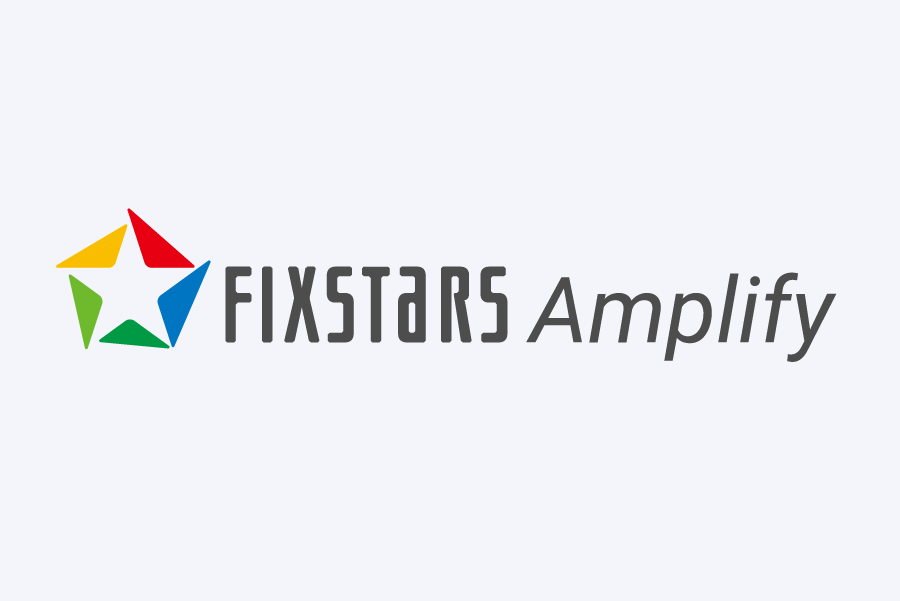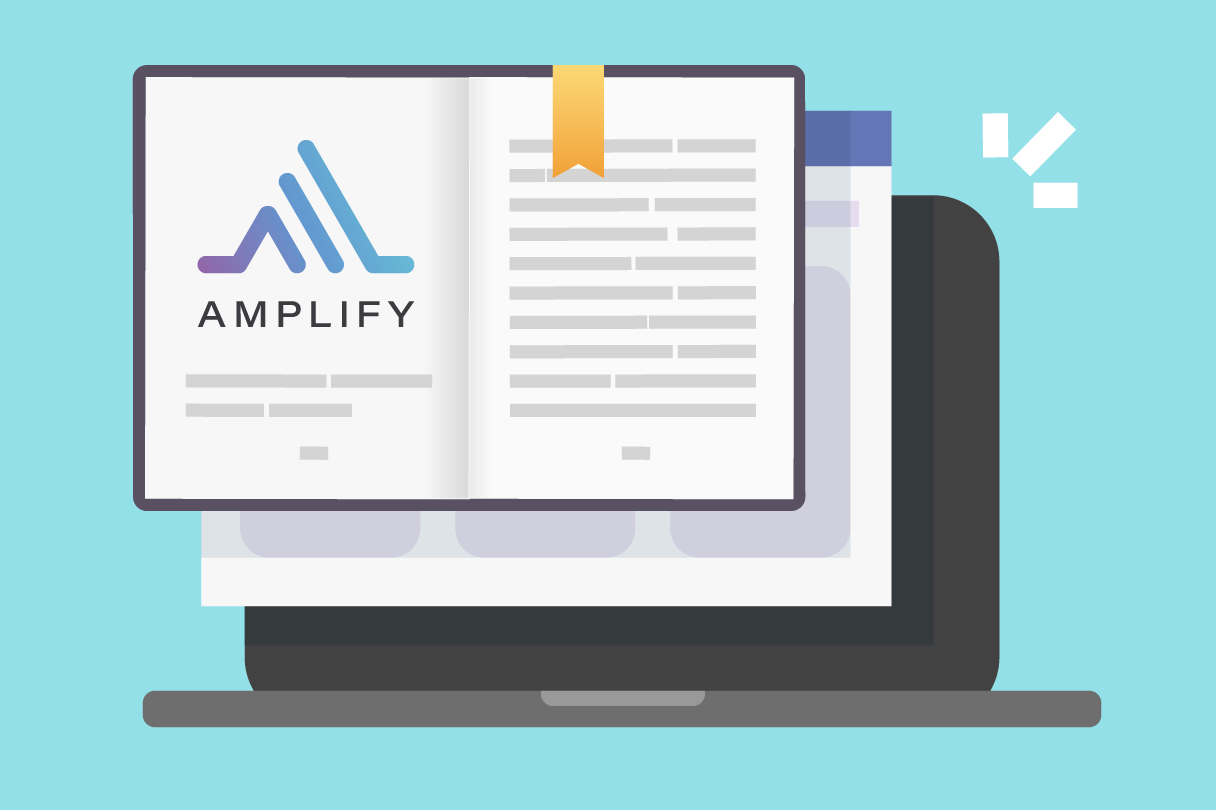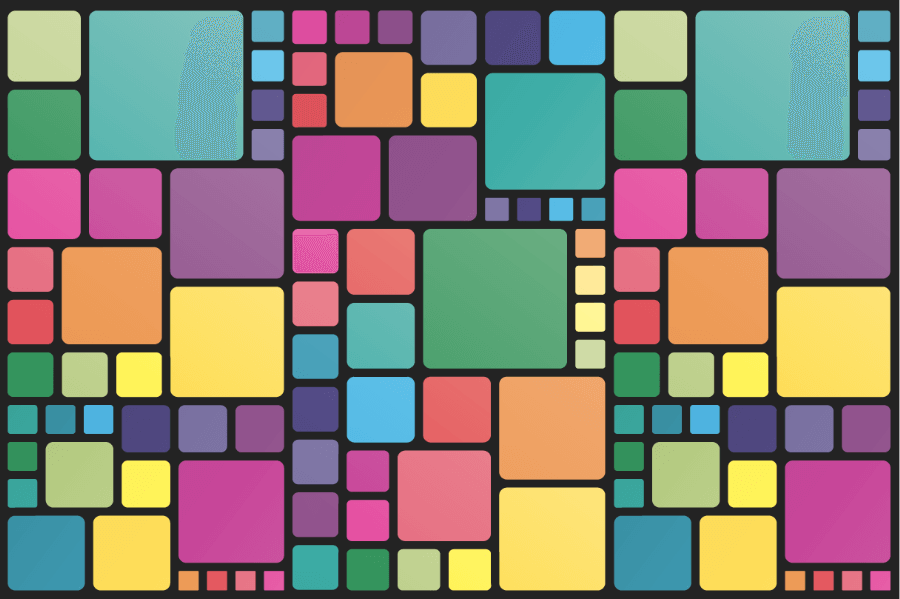# Online Demos & Tutorials

Experience Fixstars Amplify in your browser through demo applications## Experience Amplify now

You can experience Amplify applications and tutorials on your web browser.Start using Amplify SDK and AE interactively on the web based development environment below.Tutorials (basics)#### First-time users

Difficulty LevelThis is a tutorial that explains the basic programming flow of Amplify SDK.Tutorials (basics)#### Combinatorial optimization

Difficulty LevelIn this tutorial, you solve a number partitioning problem for better understanding of combinatorial optimization problems.Tutorials (basics)#### Image noise reduction

Difficulty LevelHere we develop an application that reduces noise from images.Tutorials (basics)#### Using polynomials

Difficulty LevelThis tutorial introduces Amplify features to formulate objective functions using polynoimals.Tutorials (applied)#### How to handle constraints

Difficulty LevelThis tutorial shows how to treat constraints in Amplify SDK.Tutorials (applied)#### Meeting Room Allocation Problem

Difficulty LevelIn this tutorial, we develop an application for a room allocation problem that can be formulated using constraints.Tutorials (applied)#### Taxi scheduling problem

Difficulty LevelHere we develop an application for a taxi scheduling problem that can be formulated using an objective function and constraints.Tutorials (applied)#### Using various machines

Difficulty LevelThis template contains the minimal code for running all available machines using Amplify SDK.Demo Application#### Graph coloring problem

Difficulty LevelLearn how to formulate the graph coloring problem using Fixstars Amplify.Demo Application#### Traveling Salesman Problem

Difficulty LevelLearn how to formulate the Traveling Salesman Problem using Fixstars Amplify.Demo Application#### Sudoku

Difficulty LevelLearn how to formulate Sudoku problems with Fixstars Amplify.Demo Application#### Ride Sharing

Difficulty LevelExperience an optimization application for collective ride-sharing.Demo ApplicationDifficulty LevelDemo ApplicationDifficulty Level